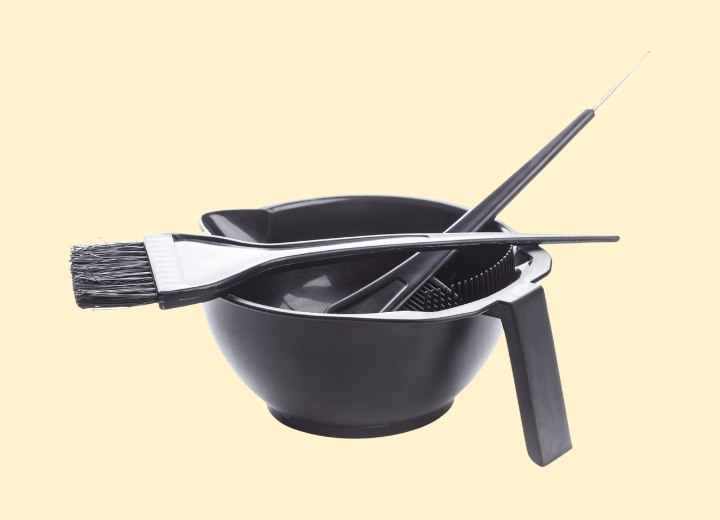# Conversion of Peroxide VolumeQ: Could you please tell me the volume of developers converted into the percentages of peroxide (eg 3% = 10vol)?

A: The easiest way to help you understand the conversions of peroxide volume to actual percentages is to explain how the process of peroxide works. When peroxide breaks down during chemical processing, it releases oxygen in specific amounts according to the strength of the peroxide in the solution.

For every 1% of peroxide in a reaction, 3.3 volumes of oxygen are released. So, if the solution of peroxide contains 3% hydrogen peroxide, its chemical decomposition will release 9.9 volumes of oxygen. We round this number up to 10.

Knowing this, we can convert the other standard developer strengths to their measure of peroxide percentages by simple division. Take the volume of the developer, and divide by 3.3. The result will give you the percentage of hydrogen peroxide in the developer.

For instance: 30-volume developer has 9.09% hydrogen peroxide in its solution. (40-volume = 12.12%; 20-volume = 6.06%; etc.) The formula for conversion does require some rounding off of the resulting numbers, but it gives you enough knowledge with which to work.

©Hairfinder.com
Photo: Photopixel/Shutterstock

See also:

How to color hair

What does the developer do?

The formula to mix peroxide